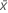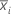# Methods and formulas for Randomization test for 1-sample mean

Select the method or formula of your choice.

## Mean

A commonly used measure of the center of a batch of numbers. The mean is also called the average. It is the sum of all observations divided by the number of (nonmissing) observations.

### Notation

TermDescription
xiith observation
Nnumber of nonmissing observations

## Standard deviation (StDev)

The sample standard deviation provides a measure of the spread of your data. It is equal to the square root of the sample variance.

### Formula

If the column contains x 1, x 2,..., x N, with mean, then the standard deviation of the sample is:

### Notation

TermDescription
x i i th observationmean of the observations
N number of nonmissing observations

## Variance

The variance measures how spread out the data are about their mean. The variance is equal to the standard deviation squared.

### Notation

TermDescription
xiith observationmean of the observations
Nnumber of nonmissing observations

## Sum

### Notation

TermDescription
xi i th observation

## Minimum

The smallest value in your data set.

## Median

The sample median is in the middle of the data: at least half the observations are less than or equal to it, and at least half are greater than or equal to it.

Suppose you have a column that contains N values. To calculate the median, first order your data values from smallest to largest. If N is odd, the sample median is the value in the middle. If N is even, the sample median is the average of the two middle values.

For example, when N = 5 and you have data x1, x2, x3, x4, and x5, the median = x3.

When N = 6 and you have ordered data x1, x2, x3, x4, x5,and x6:

where x3 and x4 are the third and fourth observations.

## Maximum

The largest value in your data set.

## Mean (bootstrap sample)

### Notation

TermDescriptionmean of the ith resample
B number of resamples
N number of observations in the original sample

## Standard deviation of the bootstrapping distribution

### Notation

TermDescriptionmean of the resamples
B number of resamplesmean of the ith resample

## P-Value

### Formula

The calculation of the p-value depends on the alternative hypothesis.
• Mean less than hypothesized value:• Mean not equal to hypothesized value:• Mean greater than hypothesized value:### Notation

TermDescription
lnumber of bootstrap means that are less than or equal to the sample mean
unumber of bootstrap means that are greater than or equal to the sample mean
βnumber of resamples
nlnumber of bootstrap means that are less than or equal to μ0d
nunumber of bootstrap means that are greater than or equal to μ0 + d
μ0hypothesized value
dmean of the observed sample
By using this site you agree to the use of cookies for analytics and personalized content.  Read our policy